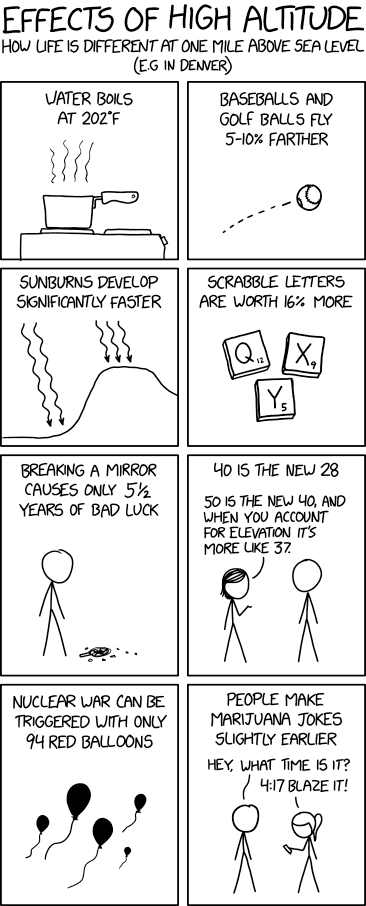assignment Homework

##### Entropy, energy, and enthalpy of van der Waals gas
Van der Waals gas Enthalpy Entropy Thermal and Statistical Physics 2020

In this entire problem, keep results to first order in the van der Waals correction terms $a$ and \$b.

1. Show that the entropy of the van der Waals gas is \begin{align} S &= Nk\left\{\ln\left(\frac{n_Q(V-Nb)}{N}\right)+\frac52\right\} \end{align}

2. Show that the energy is \begin{align} U &= \frac32 NkT - \frac{N^2a}{V} \end{align}

3. Show that the enthalpy $H\equiv U+pV$ is \begin{align} H(T,V) &= \frac52NkT + \frac{N^2bkT}{V} - 2\frac{N^2a}{V} \\ H(T,p) &= \frac52NkT + Nbp - \frac{2Nap}{kT} \end{align}Effects of High Altitude by Randall Munroe, at xkcd.

face Lecture

30 min.

##### Review of Thermal Physics
Thermal and Statistical Physics 2020

These are notes, essentially the equation sheet, from the final review session for Thermal and Statistical Physics.

face Lecture

5 min.

##### Energy and Entropy review
Thermal and Statistical Physics 2020 (3 years)

This very quick lecture reviews the content taught in Energy and Entropy, and is the first content in Thermal and Statistical Physics.